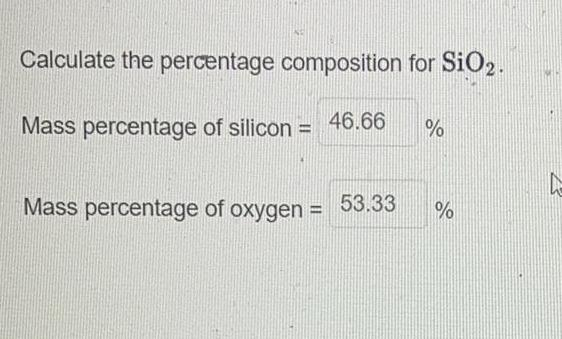Question:

# Calculate the percentage composition for SiO2. Mass

Last updated: 7/31/2022Calculate the percentage composition for SiO2. Mass percentage of silicon = ____% Mass percentage of oxygen =____%